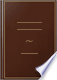Αναζήτηση Εικόνες Χάρτες Play YouTube Ειδήσεις Gmail Drive Περισσότερα »
Είσοδος
 Βιβλία Βιβλία 21 - 30 από 180 για DIVISION is the process of finding how many times one number is contained in another..DIVISION is the process of finding how many times one number is contained in another.Practical and Mental Arithmetic: Designed Principally to Accompany Daboll's ... - Σελίδα 38
των Roswell Chamberlain Smith - 1826 - 107 σελίδες
Πλήρης προβολή - Σχετικά με αυτό το βιβλίο## A Theoretical and Practical Arithmetic ...

Daniel Leach - 1857
...the excess of the number 90,845 above the number 70,767 ? DIVISION.* SECTION VI. 30. DIVISION is the process of finding how many times one number is contained in another of the same kind, or of finding into how many equal parts a given number can be divided. 31. The number to be divided is...## Introduction to The National Arithmetic: On the Inductive System : Combining ...

Benjamin Greenleaf - 1857 - 324 σελίδες
...36? In 60? In 72? In 84? In 120? In 144? ABT. 46. The pupil will now perceive that DIVISION is the process of finding how many times one number is contained in another. In division there are three principal terms : the Dividend, the Divisor, and the Quotient, or answer....## The National Arithmetic on the Inductive System: Combining the Analytic and ...

Benjamin Greenleaf - 1857 - 444 σελίδες
...times as far. How far is Neptune from the Sun ? Ans. 2,850,000,000 miles. DIVISION. 66. DIVISION is the process of finding how many times one number is contained in another ; or the process of separating a number into a proposed number of equal parts. In division there are...## A Treatise on Arithmetic, Combining Analysis and Synthesis: Adapted to the ...

James Stewart Eaton - 1857 - 355 σελίδες
...composed of 7 wires ; how many wires are there in the cable ? Ans. 133. § 6. DIVISION. DIVISION is the process of finding how many times one number is contained in another. The number to be divided is called the dividend ; the number by which to divide, the divisor ; the...## Greenleaf's Intellectual Arithmetic

Benjamin Greenleaf - 1858 - 154 σελίδες
...and 3 tons remainder ; 6 in 3 tens, or 30, 5 tunes ; answer, 15. 3. Divide 96 by 8. * DIVISION is the process of finding how many times one number is contained in another, or the process of separating a number into any proposed number of equal parts. Tho number to be divided...## The national arithmetic on the inductive system: combining the analytic and ...

Benjamin Greenleaf - 1858 - 444 σελίδες
...times as far. How far is Neptune from the Sun ? Ans. 2,850,000,000 miles. DIVISION. 66. Division is the process of finding how many times one number is contained in another ; or the process of separating a number into a proposed number of equal parts. In division there are...## The National Arithmetic on the Inductive System: Combining the Analytic and ...

Benjamin Greenleaf - 1858 - 444 σελίδες
...times as far. How far is Neptune from the Sun ? Ans. 2,850,000,000 miles. DIVISION. 661 DIVISION is the process of finding how many times one number is contained in another ; or the process of separating a number into a proposed number of equal parts. In division there are...## A Mental Arithmetic, Upon the Inductive Plan: With Easy Exercises for the ...

Benjamin Greenleaf - 1858 - 72 σελίδες
...LESSON XI. DIVISION. 1. In 24 how many times 4 ? How many times 6 ? How many times 8 ? 2. What is the process of finding how many times one number is contained in another called ? ANS. Division. 8. What is the number to be divided called ? ANS. The Dividend. 4. What is...## The Progressive Practical Arithmetic: Containing the Theory of Numbers, in ...

Horatio Nelson Robinson - 1859 - 336 σελίδες
...many times 9 in 36? in 63? in 81 ? 15. How many times 7 in 49 ? in 70 ? in 84 ? 72. Division is the process of finding how many times one number is contained in another. 73. The Dividend is the number to be divided. 74. The Divisor is the number to divide by. 75. The Quotient...## The Progressive Intellectual Arithmetic, on the Inductive Plan: Being a ...

Horatio Nelson Robinson - 1859
...and the other at the rate of 11 miles an hour, how many hours before they will meet ? Division is the process of finding how many times one number is contained in another ; and the result is called the Quotient. The sign of division is written thus, -;-, and, placed between...